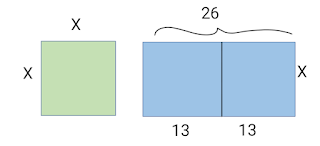# LEARNING ALGEBRA FROM A NEW PERSPECTIVE

Algebraic expressions that we see and encounter today is way different than it was centuries ago. Until 19th century, they consisted of theories. The father of algebra Muhammad ibn Musa al-Khwarizmi described algebra to be reduction and balancing of terms that is a transposition to other sides of the equation. Algebra went through different stages to development along the centuries. Along which the three stages that gives us the history of symbolic algebra are to be discussed in this article.

### 1. RHEOTORICAL STAGE

In this stage, the algebraic expressions were written in sentences. For example, an equation as: x+ 24= 36 would be described as ‘something added to 24 gives us 36’. It was developed by Babylonians back in the 16th century.

### 2. SYNCOPATED ALGEBRA

In this stage, the symbolism was used but it didn’t contain all of the characteristics of symbolic algebra, there were some restrictions involved. Syncopated algebra made its first appearance in the 3rd century AD through Diophantus Arithmetic, followed by Brahmgupta’s Brahma Sphuta Siddhanta back in the 7th century.

### 3. SYMBOLIC ALGEBRA

Symbolic algebra made its appearance through Islamic mathematicians such as Ibn al-Banna in 13-14th centuries and al-Qalasadi in 15th century in their works. And it was fully developed by Francois Viete in the 10th century. Later, Rene Descartes by 17th century, introduced the use of ‘x’ in an equation. He also showed that problem in geometry can be expressed and solved in terms of algebra. Quadratic equations played an important role in early algebra and it is to be noted that earlier only three types of quadratic equations existed:

Since math was used in commerce there were no use of negative terms. ‘p’ and ‘q’ was positive in each of the above cases and so were the roots.

On to most interesting part where mathematical expressions were solved with geometric practices. Between the rheotorical and syncopated stage of symbolic algebra, the geometric constructive algebra’ was developed by classical Greek and Vedic Indian Mathematicians in which equations were solved through geometry.

Now let’s look at this equation:

Since we already know how to find roots of quadratic equation, i.e. by using,

As they didn’t consider the negative part, let us exclude that and put the respective values, we will get

And now let’s solve it with the help of figures. Taking the x^2 as a square of side x and thus the term gives its area. And the 26x can be denoted as rectangle with sides 26 and x and the term being the area.

Now, if we divide the rectangle into two equal parts which will give us two rectangle of side x and 13.By placing the rectangles on two adjacent sides of the square, we will see a L shaped figure which can we converted into a square if we added a square of side 13 in the space.And since we added a square, we now have to add 169, which is the area of the additional square, on each sides of the equation. It gives us

The left-hand side of the equation represents the sum of areas of the 2 squares and 2 rectangle and the right-hand side gives the total area. Since all the shapes collectively makes a square, the number 246 denotes the area of the biggest square. And hence 14 will be the length of the sides. And now we know that the value of x must be 1.

While this method will only work on positive entities and will only give the positive part of the solution, we can now see the quadratic equations from a whole new perspective.